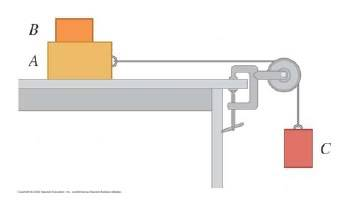# Friction and Tension problem

Hi You guys, my first post on here. I'm having a bit of a hard time getting my head around Tension and friction, so am hoping for a little help, which would be much appreciated!

## Homework Statement

Block B rests on block A, which in turn is on a horizontal tabletop. There is no friction between A and the tabletop, but the coefficient of static friction between A and B is u_s < 1. A massless string connects A to a block C. The string passes over a frictionless and massless pulley, and block C is suspended from the other end.a) draw the free-body diagrams for A+B, B and C, ( if A and B moves together )

b) what is the largest mass C can have so that A and B still slide together when the system is released from rest.?

## The Attempt at a Solution

I've drawn the free body diagrams, and trying to find a expression for m_C. But am a bit stuck. If there had been no friction bewteen A and B, B would have slid of when the system is released, so somehow the friction plays into this.
I also know that T is equal for both A and C.
And that A+B moves along the x-axis and C on the y-axis, whic means there is no a_y for A+B and no a_x for C. As they are connected they both move with the same acceleration, so far so good.

My initial thought is :

T = m_a+b*a - f and
T = w_c ( newtons 3.law, block c works on the string and the string works on block C)

and from there say
m_a+b*a - f = m_c *g (g=a)

-->

m_a+b - f/g = m_c

am I completely on the wrong track??

Astronuc
Staff Emeritus
The answer must contain $\mu_s$ and the mass of B.